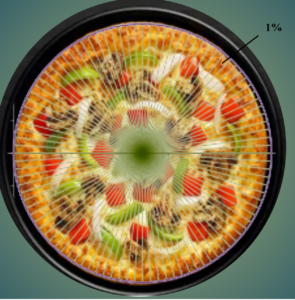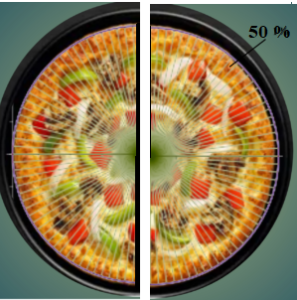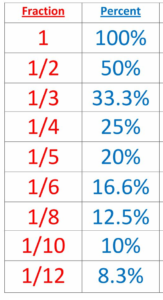December 8, 2023

# Percents with fractions: Definition, Problems and Solutions

Percents with fractions

Contents

# Percent to Fraction definition

The term “percent” is made up of two words ‘per and cent’ which means “Out of 100”. In other words, we can say that percent is the ratio calculated out of 100.  For Example , if in an examination , Rahul got  87 % marks that means Rahul has got   87 marks out of 100.  The symbol for percent  is “%” . It means “out of 100” .

Another way to visualize percent , you can take whole of something and divide it into 100 little pieces and then figure out how many little pieces are in the portion you are interested in. Far example ,If we divide a pizza into 100 little slices , then each slice would represent 1% of the pizza. If you wanted a quarter of the pizza, you would see that it would require 25 slices out of 100 or 25 % of the pizza. If you want half of pizza, it would be 50% or half of hundred percent.1% = 25%= 50% =

The percent to fraction is the conversion of percentage values to a fraction. Any percent is easy to be converted to a fraction and vice versa. The steps to convert percent into fractions are given below.

## How to Convert Percent to Fraction

Whole Number Percent to Fraction

To convert a whole number  percent into an equivalent fraction , the following steps must be followed.

Step 1. Remove the % symbol.

Step 2. Divide the number by 100.

Step 3. Reduce the fraction formed to its lowest term.

Example:  Convert 28 %    as a fraction.

Solution :  28 % = .

Mixed Number Percent to Fraction

To convert  mixed number percent to fraction, follow the steps given below.

Step 1.  Convert the  given mixed number into an improper fraction.

Step 2. Drop the percent symbol %, multiplying  by

.

Step 3.  Reduce the fraction formed to its lowest term.

Example :   Convert %  as a fraction .

Solution :

%  = % =
.

Converting Decimal Percent to Fraction

To convert decimal percent to fraction, follow the steps given below.

Step 1.  Drop the % symbol and divide it by 100.

Step 2.  Multiply both numerator and denominator by 10, 100, 1000, ………etc  , to remove the decimal point.

Step 3. Reduce the fraction formed to its lowest term.

Example :  Convert 8.5%  as a fraction.

Solution :  8.5 % = .

### “Percent to Fraction Conversion Table”

Given below is a simple percent to fraction conversion table.#### Percent to Fraction Practice Questions

Express each of the following as a fraction.

(a) 40 %

Solution:  40 % =   =

(b)  8.5 %

Solution:    8.5% =

(c) 12.5 %

Solution . 12.5% =

(d) 6.34%

Solution.  6.34% =

(e) Convert 45 % into a fraction.

Solution. 45 % =

(f) 1.25 as a fraction and percent.

Solution.   Converting 1.25 as a fraction

1.25 = =

Now,  to convert    into percent, multiply

by 100.

= 125%

(g) 0.25 as a fraction and percent

Solution:   Converting 0.25 as a fraction

0.25 =

=

Now ,  to convert

into percent , multiply   by 100.

= 25%

(h) 57 % as a fraction.

Solution : 57% =

(i)  0.125 as a fraction and percent.

Solution:  Converting 0.125 as a fraction

0.125 =

= =

Now,  to convert    into percent, multiply

by 100.

= 12.5%

(j)7.5 % as a fraction.

Solution :  7.5 % =

.

(k)66.6 % as a fraction.

Solution:  66.6 % =

(l)3 % as a fraction

Solution :   3% =

##### Percent to Fraction Worksheet [PDF]

You must be also interested in:#### Bina singh

View all posts by Bina singh →
Last Minute Preparation Tips for SSC CGLTier II 2023 ssc chsl 2023 tier 1 cut off NIRF Rankings 2023 : Top 10 Engineering colleges in India CBSE Compartment Exam 2023 Application Form SSC CGL 2023 Notification: Important Dates